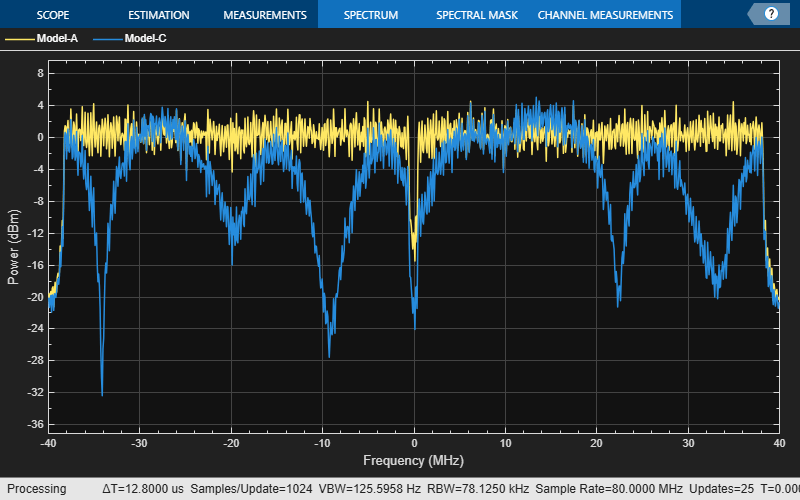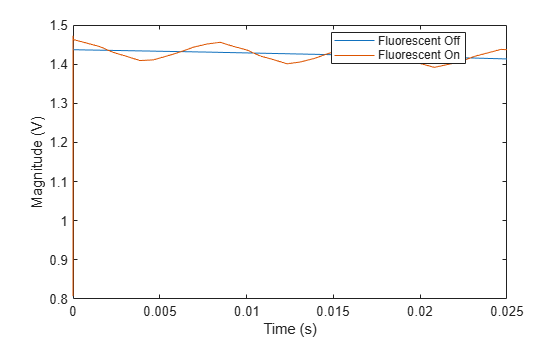Main Content

# Delay Profile and Fluorescent Lighting Effects

This example demonstrates the impact of changing the TGac delay profile, and it shows how fluorescent lighting affects the time response of the channel.

### Delay Profile Effects

Create VHT configuration object. Set the sample rate to 80 MHz.

```cfgVHT = wlanVHTConfig; fs = 80e6;```

Generate random binary data and create a transmit waveform parameterized by the VHT configuration object.

```d = randi([0 1],8*cfgVHT.PSDULength,1); testWaveform = wlanWaveformGenerator(d,cfgVHT);```

Create a TGac channel object. Set the delay profile to `'Model-A'`, which corresponds to flat fading. Disable large-scale fading effects.

```tgacChan = wlanTGacChannel('SampleRate',fs, ... 'ChannelBandwidth',cfgVHT.ChannelBandwidth, ... 'DelayProfile','Model-A', ... 'LargeScaleFadingEffect','None');```

Pass the transmitted waveform through the TGac channel.

`rxModelA = tgacChan(testWaveform);`

Set the delay profile to Model-C, which corresponds to a multipath channel with 14 distinct paths and a 30 ns RMS delay spread. The maximum delay spread is 200 ns, which corresponds to a coherence bandwidth of 2.5 MHz.

```release(tgacChan) tgacChan.DelayProfile = 'Model-C';```

Pass the waveform through the model-C channel.

`rxModelC = tgacChan(testWaveform);`

Create a spectrum analyzer and use it to visualize the spectrum of the received signals.

```saScope = dsp.SpectrumAnalyzer('SampleRate',fs, ... 'ShowLegend',true,'ChannelNames',{'Model-A','Model-C'}, ... 'AveragingMethod','Exponential','ForgettingFactor',0.99); saScope([rxModelA rxModelC])```As expected, the frequency response of the model-A signal is flat across the 80 MHz bandwidth. Conversely, the model-C frequency response varies because its coherence bandwidth is much smaller than the channel bandwidth.

### Fluorescent Effects

Release the TGac channel, and set its delay profile to `'Model-D'`. Disable the fluorescent lighting effect.

```release(tgacChan) tgacChan.DelayProfile = 'Model-D'; tgacChan.FluorescentEffect = false;```

To better illustrate the Doppler effects of fluorescent lighting, change the bandwidth and sample rate of the channel. Generate a test waveform of ones.

```tgacChan.ChannelBandwidth = 'CBW20'; fs = 20e6; tgacChan.SampleRate = fs; testWaveform = ones(5e5,1);```

To ensure repeatability, set the global random number generator to a fixed value.

`rng(37)`

Pass the waveform through the TGac channel.

`rxSig0 = tgacChan(testWaveform);`

Enable the fluorescent lighting effect. Reset the random number generator, and pass the waveform through the channel.

```release(tgacChan) tgacChan.FluorescentEffect = true; rng(37) rxSig1 = tgacChan(testWaveform);```

Determine the time axis and channel filter delay.

```t = ((1:size(rxSig0,1))'-1)/fs; fDelay = tgacChan.info.ChannelFilterDelay;```

Plot the magnitude of the received signals while accounting for the channel filter delay.

```plot(t(fDelay+1:end),[abs(rxSig0(fDelay+1:end)) abs(rxSig1(fDelay+1:end))]) xlabel('Time (s)') ylabel('Magnitude (V)') legend('Fluorescent Off','Fluorescent On','location','best')```Fluorescent lighting introduces a Doppler component at twice the power line frequency (120 Hz in the U.S.).

Confirm that the peaks are separated by approximately 0.0083 s (inverse of 120 Hz) by measuring distance between the second and third peaks.

```[~,loc] = findpeaks(abs(rxSig1(1e5:4e5))); peakTimes = loc/fs; peakSeparation = diff(peakTimes)```
```peakSeparation = 0.0085 ```

## SupportGet trial now